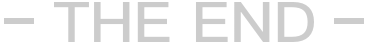if(\$CrossID=='') \$orwheres[] = ' arc.typeid IN ('.GetSonIds(\$typeid).')';

else \$orwheres[] = ' arc.typeid IN ('.GetSonIds(\$typeid).','.\$CrossID.')';

if(\$CrossID=='') \$orwheres[] = ' (arc.typeid IN ('.GetSonIds(\$typeid).') OR arc.typeid2 IN ('.GetSonIds(\$typeid).')) ';

else \$orwheres[] = ' (arc.typeid IN ('.GetSonIds(\$typeid).','.\$CrossID.') OR arc.typeid2 IN ('.GetSonIds(\$typeid).','.\$CrossID.')) ';